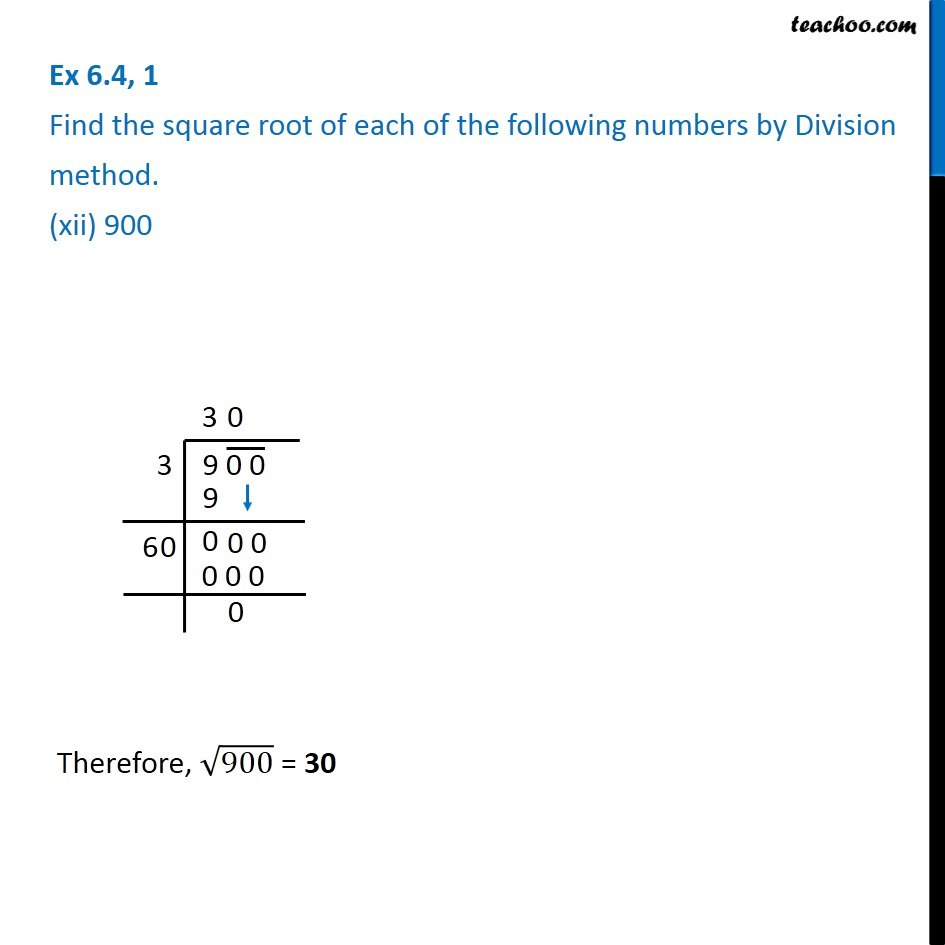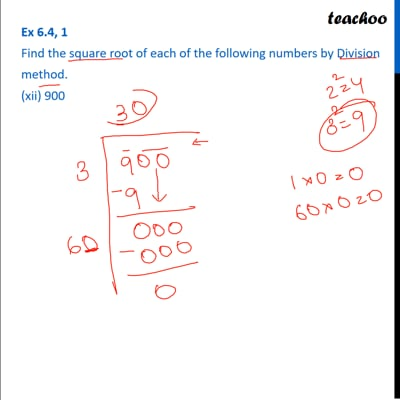Ex 6.4

Chapter 6 Class 8 Squares and Square Roots
Serial order wiseThis video is only available for Teachoo black users

Get live Maths 1-on-1 Classs - Class 6 to 12

### Transcript

Ex 6.4, 1 Find the square root of each of the following numbers by Division method. (xii) 900 Individual Steps are explained Step 1: Write the number We make pairs from right. So, 04 and 23 are two pairs. Step 2: Now, we find that number whose square is less than or equal to 23. 3 × 3 = 9 4 × 4 = 16 5 × 5 = 25 Since 25 > 23, we use 4 × 4 = 16 Therefore, √900 = 30